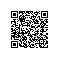# [Node.js源码解读(3)]Buffer的8KB池分配规则和固定位数字的读写

## 8KB 池分配规则

• new Buffer() （已不推荐使用，可能会泄露内存中潜在的敏感信息，具体例子可以看这里
• Buffer.alloc()
• Buffer.allocUnsafe()（虽然也有泄露内存中敏感信息的可能，但语义上非常明确）
• Buffer.from()
• Buffer.concat()

// lib/buffer.js
// ...

Buffer.poolSize = 8 * 1024;
var poolSize, poolOffset, allocPool;

function createPool() {
poolSize = Buffer.poolSize;
allocPool = createBuffer(poolSize, true);
poolOffset = 0;
}
createPool();

function createBuffer(size, noZeroFill) {
flags[kNoZeroFill] = noZeroFill ? 1 : 0;
try {
const ui8 = new Uint8Array(size);
Object.setPrototypeOf(ui8, Buffer.prototype);
return ui8;
} finally {
flags[kNoZeroFill] = 0;
}
}

function allocate(size) {
if (size === 0) {
return createBuffer(size);
}
if (size < (Buffer.poolSize >>> 1)) {
if (size > (poolSize - poolOffset))
createPool();
var b = allocPool.slice(poolOffset, poolOffset + size);
poolOffset += size;
alignPool();
return b;
} else {
return createBuffer(size, true);
}
}

// lib/buffer.js
// ...

Buffer.alloc = function(size, fill, encoding) {
// ...
return createBuffer(size);
};

Buffer.allocUnsafe = function(size) {
assertSize(size);
return allocate(size);
};

Buffer.from = function(value, encodingOrOffset, length) {
// ...
if (value instanceof ArrayBuffer)
return fromArrayBuffer(value, encodingOrOffset, length);

if (typeof value === 'string')
return fromString(value, encodingOrOffset);

return fromObject(value);
};

function fromArrayBuffer(obj, byteOffset, length) {
byteOffset >>>= 0;

if (typeof length === 'undefined')
return binding.createFromArrayBuffer(obj, byteOffset);

length >>>= 0;
return binding.createFromArrayBuffer(obj, byteOffset, length);
}

function fromString(string, encoding) {
// ...
if (length >= (Buffer.poolSize >>> 1))
return binding.createFromString(string, encoding);

if (length > (poolSize - poolOffset))
createPool();
var actual = allocPool.write(string, poolOffset, encoding);
var b = allocPool.slice(poolOffset, poolOffset + actual);
poolOffset += actual;
alignPool();
return b;
}

Buffer.concat = function(list, length) {
// ...
var buffer = Buffer.allocUnsafe(length);
// ...
return buffer;
};

• 通过 Buffer.allocUnsafeBuffer.concatBuffer.from（参数不为一个 ArrayBuffer 实例）和 new Buffer（参数不为一个 ArrayBuffer 实例）创建。
• 传入的数据大小不为 0 。
• 且传入数据的大小必须小于 4KB 。

## 那些固定位数字读写 API

• 没有显著的性能提升。
• 会在实例被初始化后又增加新的属性。
• noAssert 参数将会失效。

Buffer.prototype.writeUInt32BE = function(value, offset, noAssert) {
value = +value;
offset = offset >>> 0;
if (!noAssert)
checkInt(this, value, offset, 4, 0xffffffff, 0);
this[offset] = (value >>> 24);
this[offset + 1] = (value >>> 16);
this[offset + 2] = (value >>> 8);
this[offset + 3] = value;
return offset + 4;
};

Buffer.prototype.readUInt32BE = function(offset, noAssert) {
offset = offset >>> 0;
if (!noAssert)
checkOffset(offset, 4, this.length);

return (this[offset] * 0x1000000) +
((this[offset + 1] << 16) |
(this[offset + 2] << 8) |
this[offset + 3]);
};

## 最后使用钉钉扫一扫加入圈子
+ 订阅